# Learn Addition Using a Number Line

1. Math Lessons >

### Overview of Addition using a Number Line:

Have you been looking for a simple tool that helps ease you into adding numbers? Then, the number line is your best bet. In this lesson, we'll show you how to add numbers on a number line.

### How Do We Add Numbers on a Number Line?

Let’s get started with 4 + 3. We have Mr. Lollihops waiting for us on the number line.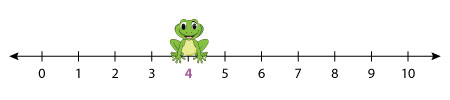Click to watch him swing into action.

As you can see, he starts from 4, jumps 3 steps forward, and lands on 7.

So, we can say 4 + 3 = 7.

### What Happens When Addends Change Positions?

Let’s now find 2 + 8. We have Mr. Boomer ready to hop on!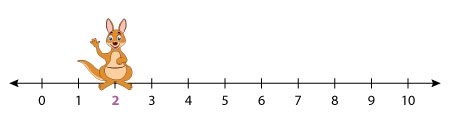Animate him with a click!

Mr. Boomer starts at 2, and hops 8 steps forward, and lands on 10.

So, we say 2 + 8 = 10.

But what if Boomer starts at 8? Then, he will have to hop 2 steps forward.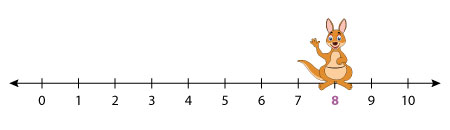Click to see him hopping.

Did you notice that? 2 + 8 and 8 + 2 both equal 10.

Thus no matter which order the numbers are added in, the sum remains the same.

### Let’s Add Three Numbers This Time!

What if you’re up against three addends? Don’t be tripped up! Just continue to hop for the third addend from the point where the hops for the second addend stop. Here’s our number line that models 5 + 4 + 6.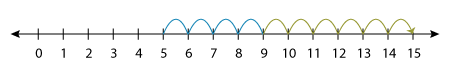As you can see, we started with 5, made 4 hops to land at 9, and then drew 6 more hops from there to obtain a sum of 15.

So, our addition sentence is “5 + 4 + 6 = 15”.

Thus, you can go on hopping for every number that is added.

### How Do We Find a Missing Addend Using a Number Line?

Observing the above number line models, we can conclude three things:

Draw as many hops as the other addend.

Land at the sum.

Thus, we can easily figure out any missing addend by simply counting the hops or with the start point. Let’s take this model for instance and complete the addition sentence: 6 + _____ = 11.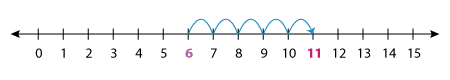How many hops are drawn? The answer to this question will give you the missing addend.

Yes, you’re right! It’s 5.

The addition equation is “6 + 5 = 11”.

1) Which addition sentence does the number line represent?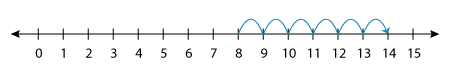The number line represents 8 + 6 = 14.

2) What is 5 + 4 + 3? Find the sum using a number line diagram.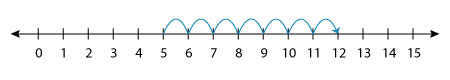Thus, we have 5 + 4 + 3 = 12.

3) Which number is added to 9 so we get 15?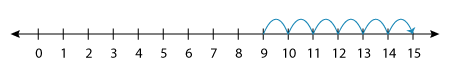We get 15 when we add 6 to 9.